# Year 3 Multiplication Practice Questions | 10 Problems to Test Your Child

Multiplication is a fundamental concept that every student needs to master in order to do well maths. So, we've prepared 10 multiplication practice questions with solutions for Year 3 students to test their skills.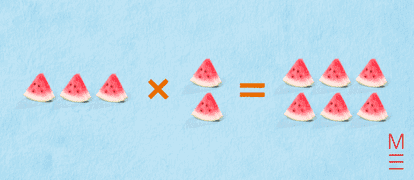Can you solve these 10 Year 3 multiplication practice questions? No calculators allowed!

## 10 Year 3 multiplication practice questions to test your child

These 10 Year 3 multiplication practice questions are assessable under NESA’s current Mathematics Syllabus. You can find the Year 3 Maths syllabus for 2022 here. Please note that a new Year 3 Maths syllabus will be implemented from 2023. You will be able to find the new syllabus on the NESA website when it is available. If you want to learn more about NAPLAN and how to prepare for it, read our article here.

## Multiplication Practice Question 1

Nancy went to the aquatic centre. She swam 5 laps in the outdoor 50-metre pool before she felt cold and went inside to swim 4 laps in the 25-metre pool. How far did she swim in total?

A) 250 m

B) 350 m

C) 450 m

D) 100 m

### Solution:

The correct answer is B) 350 m.

In the 50-metre pool, Nancy swam 5 laps, which is a distance of:

$$50 \times 5 = 250 \text{ m}$$

In the 25-metre pool, Nancy swam 4 laps, which is a distance of:

$$25 \times 4 = 100 \text{ m}$$

So, in total, Nancy swam:

$$250+100 = 350 \text{ m}$$

## Multiplication Practice Question 2

When Tiffany arrives at school in the morning, she realises that she left her homework at home. Her mum drives back home to get her homework and then drops it off at school. After this whole morning fiasco, Tiffany’s mum finally drives back home to relax. If Tiffany’s house is 8 km from school, how far did her mum travel in total?

A) 24 km

B) 28 km

C) 30 km

D) 32 km

### Solution:

The correct answer is D) 32 km.

Let’s list out each time when Tiffany’s mum made a trip to/from school:

1. Drive to school
2. Return home to get the homework
3. Drive to school again
4. Return back home

So, Tiffany’s mum drove between school and home 4 times in total. There is 8 km between school and home.

That means that Tiffany’s mum drove a total distance of:

$$8 \times 4 = 32 \text{ km}$$

## Multiplication Practice Question 3

Tracey lays out her money, as shown below.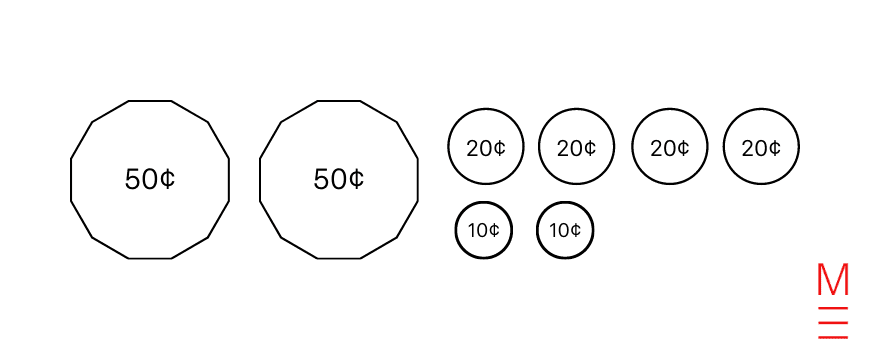A) $3.00How much money does Tracey have? B)$1.50

C) $1.80 D)$2.00

### Solution:

The correct answer is D) $2.00. There are: • 2 x 50¢ = 100¢ =$1.00
• 4 x 20¢ = 80¢ = $0.80 • 2 x 10¢ = 20¢ =$0.20

So, Tracey has a total of:

$$1.00 + 0.80 + 0.20 = 2.00$$

## Multiplication Practice Question 4

Jerry is trying to figure out how many pastries he needs to bake for his party. He has invited 13 guests, and he wants to make 3 pastries for each person in attendance, including himself. Hence, how many pastries does Jerry need?

A) 42

B) 39

C) 44

D) 40

### Solution:

The correct answer is A) 42.

There will be a total of 14 people at Jerry’s party (13 guests plus himself).

To provide each of the 14 people with 3 pastries, Jerry will need to make:

$$14 \times 3 = 42 \text{ pastries}$$

## Multiplication Practice Question 5

Anne likes to cut her square sandwiches into two equally-sized triangles. The diagram below shows what this looks like.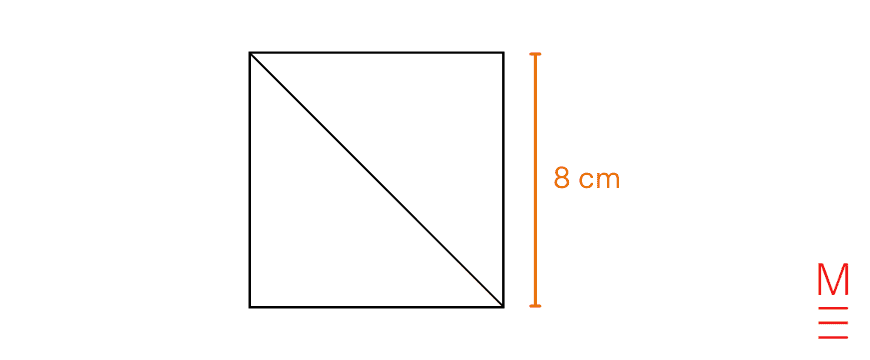What is the area of a triangular slice?

A) 16 cm2

B) 30 cm2

C) 32 cm2

D) 64 cm2

### Solution:

The correct answer is C) 32 cm2.

The area of the triangle:

\begin{align*}
\frac{1}{2} \times 8 \times 8 &= \frac{1}{2} \times 64 \\
&= 32 \text{ cm}^2 \\
\end{align*}

## Multiplication Practice Question 6

New carpet is to be laid out in a library. It is to be laid out over the whole floor, except where there are shelves. The library covers an area of 35 m2 and each shelf covers 5 m2. If there are three shelves (as shown in the arrangement below), how many square metres of carpet are required?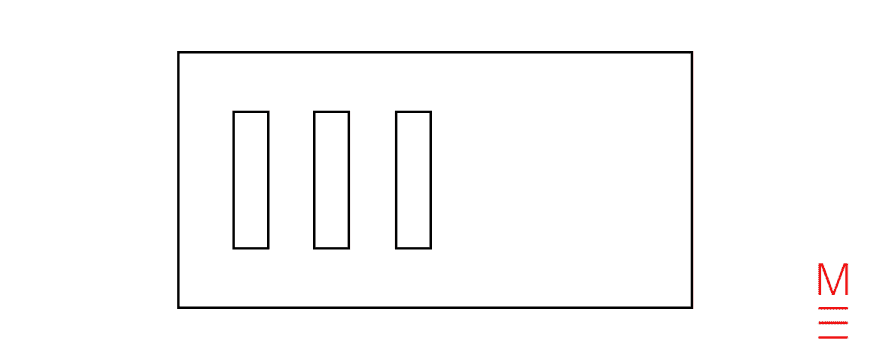A) 20 m2

B) 25 m2

C) 30 m2

D) 35 m2

### Solution:

The correct answer is A) 20 m2.

Area of carpet required:

\begin{align*}
35 – 5 \times 3 &= 35 – 15 \\
&= 20 \text{ m}^2 \\
\end{align*}

## Multiplication Practice Question 7

At the aquarium, Gilbert sees a big tank. The dimensions are shown below: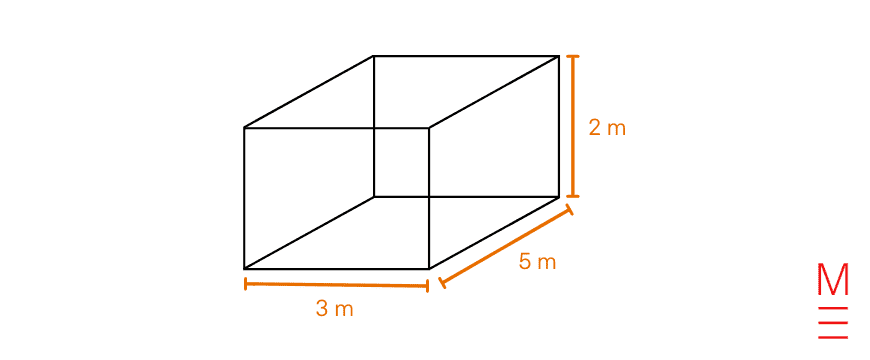What is the volume of the tank?

A) 20 m3

B) 27 m3

C) 28 m3

D) 30 m3

### Solution:

The correct answer is D) 30 m3.

Volume of the tank:

\begin{align*}
3 \times 5 \times 2 &= 15 \times 2\\
&= 30 \text{ m}^3\\
\end{align*}

## Multiplication Practice Question 8

Maurice wants to paint her orange wall. Using the diagram below, what is the area of the orange wall?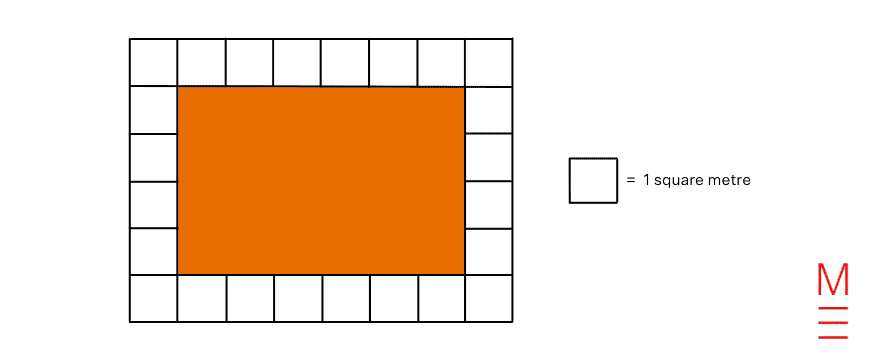A) 20 m2

B) 24 m2

C) 30 m2

D) 34 m2

### Solution:

The correct answer is B) 24 m2.

Using the scale in the diagram, the length of the wall is 6 metres and the width of the wall is 4 metres.

So, the area of the wall is:

$$6 \times 4 = 24 \text{ m}^2$$

## Multiplication Practice Question 9

Nick has 17 empty pots right now, but he wants to grow tomatoes in each of the pots. If he intends to plant 9 seeds in each pot, how many tomato seeds does he need?

A) 90

B) 121

C) 153

D) 170

### Solution:

The correct answer is C) 153.

$$17 \times 9 = 153 \text{ seeds}$$

## Multiplication Practice Question 10

Alex is trying to choose his outfit for the day. He has 3 different ties, 7 different shirts, 5 different pants and 2 different pairs of shoes. How many different outfit combinations can he wear?

A) 150

B) 180

C) 200

D) 210

### Solution:

The correct answer is D) 210.

The different outfit combinations can be represented with the following diagram: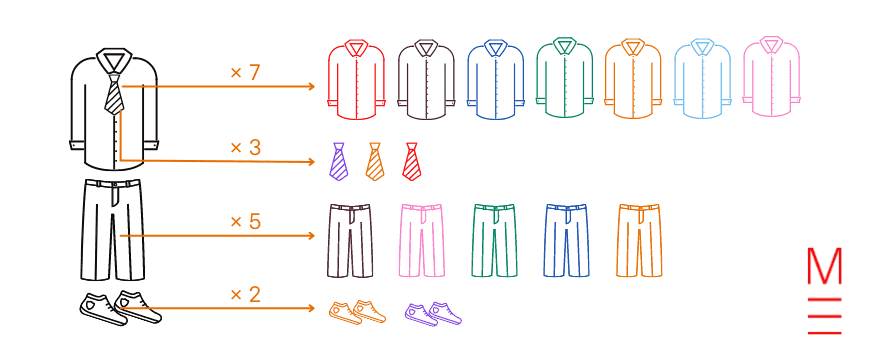By referring to the diagram, we can conclude that the total number of possible outfit combinations is:

\begin{align*}
3 \times 7 \times 5 \times 2 &= (3 \times 7) \times (5 \times 2) \\
&= 21 \times 10 \\
&= 210 \\
\end{align*}

### Get free study tips and resources delivered to your inbox.

Join 75,893 students who already have a head start.

Our website uses cookies to provide you with a better browsing experience. If you continue to use this site, you consent to our use of cookies. Read our cookies statement.